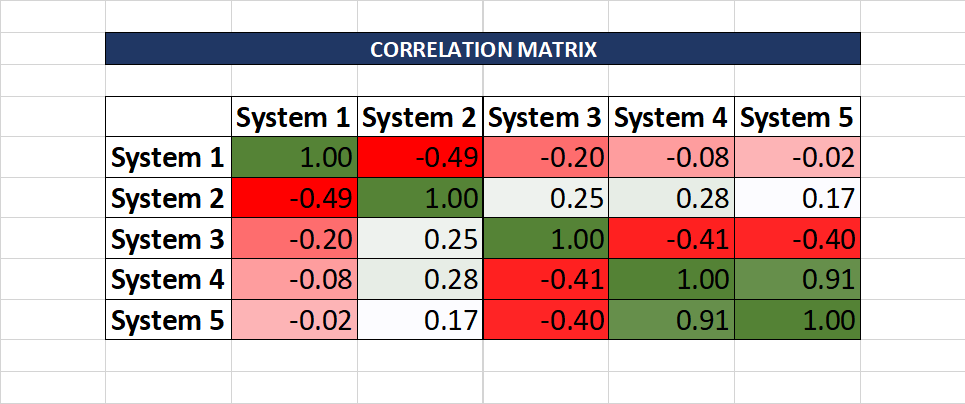Most of the modern Algorithmic traders use a combination of trading systems to generate Alpha consistently. They do implement multiple systems to make sure that the risk is diversified and they are well protected against any black swan events. For example: combining non-directional systems with directional systems provides some cushioning during volatile or sideways markets.

If you also want to use multiple trading systems, it’s important that you make sure that the systems are not heavily correlated as it would defeat the purpose. In this post, we’ll learn how to create a correlation matrix among different systems to understand how similar are those systems. The correlation of trading systems would be calculated based on their net returns, however, you can also use other parameters such as Drawdowns, Sharpe ratio, Expectancy, and so on.

A Spreadsheet is attached at the end of this post which serves as a template to calculate the correlation matrix of trading systems within a click of a button.

Correlation Matrix of Stocks in a Spreadsheet – Automatic Data Feed

The spreadsheet will serve as a one-click solution to derive the correlation matrix of trading systems.

See below the screenshot of the spreadsheet:For columns A to F in the spreadsheet, you’ll need to enter the monthly net returns of the different systems you have implemented.

And based on what you enter the correlation matrix would be automatically calculated on the right-hand side. The matrix is color-coded, and the colors range from dark red (for -1 value) to dark green (for +1 value).

If you click on any cell within the correlation matrix, you will find the below formula that is used to calculate the correlation coefficient

`=correl(\$E:\$E,F:F)`

The formula takes two inputs:

Input 1: Array of values for system 1

Input 2: Array of values for system  2

## Interpretation of Correlation Matrix

Let’s take an example of the below correlation matrix:As a general rule, avoid two systems that are highly correlated to each other, otherwise, you won’t diversify your risks.

For example- System 4 and System 5 above have a correlation coefficient of 0.91 which is pretty high. It essentially means that 1% of profit in System 4 may lead to 0.91% profit in System 5, the same applies for losses too. So there is no real reason to trade two similar systems, instead, you can double your positions in one of the systems.

Now, look at System 1 and System 2, their correlation coefficient is -0.49, which implies they are negatively correlated. So when you make a 100\$ profit on system 1, it may cause 49\$ loss on system 2 and vice versa. This reduces your overall profit but mitigates the risks too.

Also remember, that if the overall projected returns of any system is negative, then there is no reason to add that system to the correlation matrix. Because the system would be in a loss irrespective of its correlation with other systems.